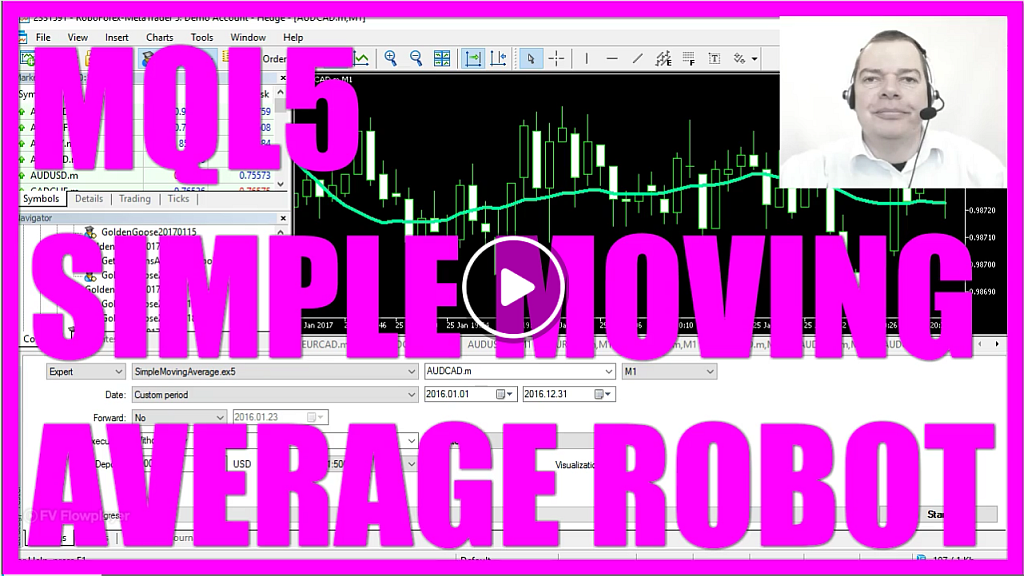If you have been trading for a while, chances are high that you have used or maybe you have seen a moving average.
This is a moving average and it is a line and you can use it to find out if the current price is above or below the average value of a particular number of candles.
This is a moving average for the last twenty candles and if you right click on it, you can change the properties here.
So let’s change the period to one and now you see that the moving average for one candle is connecting all the closed prices.
This is a bearish candle so it closes here. This is a bullish candle so here is the closing price.
Let’s look again at the properties and select one hundred and now you don’t even see the moving average because it’s far above the candles.
As traders we use moving averages to find out if it’s going down and that would be the case when the current prices crosses the moving average from the above.
Or if it’s going up and that would be the case when the current price crosses the moving average from below.
Now how can we create an Expert Advisor for this? Now if you point your mouse on any point of the moving average, you will see a value and you will see that the value would change with very candle.
Here it is 0.988749 and the closing price is 0.98848.
So let’s create an Expert Advisor for that, to do that please hit this little button here or press the F4 key to bring up the Meta Editor.
Now you need to click on new Expert Advisor from template, continue. We will give it the name simple moving average, continue, continue, finish and remove everything above the OnTick function.
Let’s remove the two comment lines and the first thing we need is an array to hold our price values.
So we will create an array with the name my moving average array and in the next step we need to define how the moving average should look like.
We want to give it the name moving average definition and it should be for the current symbol on the chart and for the current used period on the chart.
I would like to use the last twenty candles for the calculation. I don’t want to shift it to the right or to the left direction and it should be a simple moving average.
That’s what MODE_SMA does for closed price of the candle.
Now that we have defined how our simple moving average should look like, we need to sort the price range from the current candle downwards.
This is done with arraysetasseries, we do it for the moving average array.
And now we want to fill it with prize data, that is done with copy buffer for the defined EA moving average definition.
It has only one line so we places a zero here, we want the current candle, this is another zero and we want to copy the prize for three candles so let’s put in a three.
And MQL 5 should store the results in the my moving average array. So let’s calculate the current value by assigning the value of my moving average array for the current candle to the my moving average value and the last thing we want to do is to output the current EA on the chart so we use the comment function to print out the text moving average value and the calculated value we created here.
Okay let’s compile the Expert Advisor, click this little button or hit the F4 key.
And now we want to do a little trick, we now want to insert an indicator for the trend and it should be a moving average and for our calculation we use the twenty candles period, a shift value of zero.
It should be a simple moving average and it should be applied to the close price. This is the color I want to choose for the A and I want to have these kinds of lines.
So let’s click on okay and here is our visual representation.
Now we right click on the chart, go to templates and save the template as tester.tpl because this is the template the Strategy Tester is going to use, when we test our little Expert Advisor.
Bring up the Strategy Tester by choosing it from the menu or clicking control and R, select the simple moving average.ex5 file, mark the visualisation option and start the test.
And here is how it looks like.
Let’s pause the test here and our calculated value is 0.993984 and this is exactly what the current Expert Advisor value for the moving average on a chart currently is.
Continue, now it’s 0.994416, well let’s check the current value here and it’s 0.9994416. So it works!
Now you know, how you can calculate the current moving average by creating this little Expert Advisor.